• MATH
• ENGLISH
• SCIENCE
• KNOWLEDGE HUB

Topics: math

# Math Lessons Support Student Discourse

November 10, 2022every child is capable of greatness.

Posted in: Aha! Blog > Eureka Math Blog > math > Math Lessons Support Student Discourse

Anyone who reads or experiences Eureka Math2 can see the importance we place on math discourse. Our lessons revolve around rich classroom discussions that invite all learners to express and understand complex mathematical concepts. Leading these kinds of discussions isn’t easy, but Eureka Math2 helps teachers create rich mathematical discourse by giving them tools like open-ended lessons, guidance for instructional support and differentiation, and student work samples with correct and incorrect strategies.

## Five Practices for Orchestrating Productive Mathematics Discussions

Our approach to supporting teachers in creating these discussions is informed by the book Five Practices for Orchestrating Productive Mathematics Discussions (2011) by Margaret S. Smith and Mary Kay Stein. Published by the National Council of Teachers of Mathematics (NCTM), Five Practices outlines a method that teachers can use to achieve their intended instructional goals through rich classroom discussions guided by students’ ideas.

The five practices of anticipating, monitoring, selecting, sequencing, and connecting represent a unified teaching sequence of careful planning, thoughtful observation, and logical presentation of student work. The book aligns with the NCTM’s recommendations for effective teaching in its 2014 Practices to Actions, and its approach is similar to Cognitively Guided Instruction (Carpenter et al. 2014) and the bansho (Kuehnert et al. 2018) method.

Five Practices follows the research-based educational theory of social constructivism, which states that “complex knowledge and skills are learned through social interactions” (Smith and Stein 2011, 1). According to this theory, each of us constructs our own knowledge by interacting with others, either through collaboration or with guidance from someone who knows a little more than we do. It’s the theory that gave us the idea of zone of proximal development (ZPD), which places the ideal level of instruction in between what a child knows independently and what they could learn with the help of a teacher or more advanced peers (Vadeboncoeur and Rahal 2013). Social constructivism also supports instructional strategies like small-group discussions and turn and talk.

We’ll look at each of the five practices in a series of articles that will show you where you can find each practice in Eureka Math2. And you’ll read about the evidence-based principles underlying the five practices, which we hope will give you an even better appreciation for our innovative, accessible, and research-based curriculum.

## 1. Anticipating

The first practice for orchestrating productive mathematics discussions is “anticipating students’ solutions to a mathematics task” that is complex enough to generate high-level student thinking and multiple solution paths (Smith and Stein 2011, 7). When students explain their solutions in a well-orchestrated class discussion, the teacher must balance eliciting student discourse with achieving the goals of the lesson (Smith and Stein 2011, 2). Allowing too much unstructured student discourse risks having a discussion with no clear resolution, but overemphasizing lesson goals focuses the discussion on teacher explanation instead of student exploration. So preparing for a well-orchestrated discussion begins with anticipating what ideas students might present and considering how those ideas align to the lesson’s goals.

Anticipating happens during lesson preparation, which Smith and Stein say is an essential part of implementing the five practices (Smith and Stein 2011, 7). The Eureka Math2 Teach book helps teachers to think about students’ solution paths by using overviews and the Why sections to explain the mathematics covered in the upcoming topic, module, and lessons. The overviews and the Why section describe the mathematical coherence and key understandings. They also provide insights about different ways to approach problems as well as the efficiencies of various strategies and models. The information within the overviews and the Why section allows teachers to understand how the lessons relate to each other and to anticipate the thinking and work that students are likely to produce as they move through each one.

Once they understand the overall purpose of the math and their students’ potential strategies, teachers work through the problems that their students will solve and discuss in class. Beyond just finding the answer, teachers need to think about the multiple solution paths that their students might take. They should consider the ways that they can guide students who choose those paths, including how to address incorrect or misapplied strategies (Smith and Stein 2011, 8, 36). Smith and Stein (2011) recommend that teachers make a chart of these different paths (9). The other articles in this series will explain more about this chart and how helpful it is to a well-orchestrated discussion.

The authors of Five Practices also point out that the complex instructional task required for a rich mathematical discussion should engage all students (Smith and Stein 2011, 15–19). To help teachers achieve this goal, each Eureka Math2 lesson highlights at least one low-floor, high-ceiling task, eliminating the challenging step of task selection or creation for teachers, so that  rich and equitable mathematical discussion can blossom. For students who are English learners or not yet fluent readers, the concepts in Eureka Math2 lessons are supported by digital interactives, digital lessons, and wordless context videos.

Let’s look at an example of anticipation at work in grade 5 module 2 lesson 14. The lesson is about applying different methods to subtracting mixed numbers, an idea that opens the door wide to multiple solution paths. At a glance, teachers can see several approaches (purple) listed in the Topic Overview, as well as a through line (blue) that explains the lesson’s purpose in the story of the topic:

Students find sums and differences in expression involving mixed numbers. To add, students use a variety of methods, such as the next whole, adding like units (i.e., whole numbers with whole numbers and fractions with fractions), and renaming fractions greater than 1 as mixed numbers. To subtract, students decompose the minuend to take from 1 or decompose the subtrahend. They represent their work by using a number line, the arrow way, and a number bond. Throughout the topic, students are encouraged to try different methods so they can make an appropriate choice based on the numbers in a given expression. Tough all methods are validated and acceptable, students should notice that some methods can be more efficient than others. Students continue to estimate a sum or a difference to check the reasonableness of their answers.

Once teachers look at the first problem, they can see that students can approach it in diverse ways. That’s because the prompt guides students to draw a model but doesn’t dictate the method:

Use the Read-Draw-Write process to solve the problem.

1. Yuna's car trip will take 923 hours of driving. She stops to take a break after 545 hours.
How many hours does Yuna still need to drive after her break?

And in case the teachers have missed any solution paths, the next page of Teach provides them with some sample student work.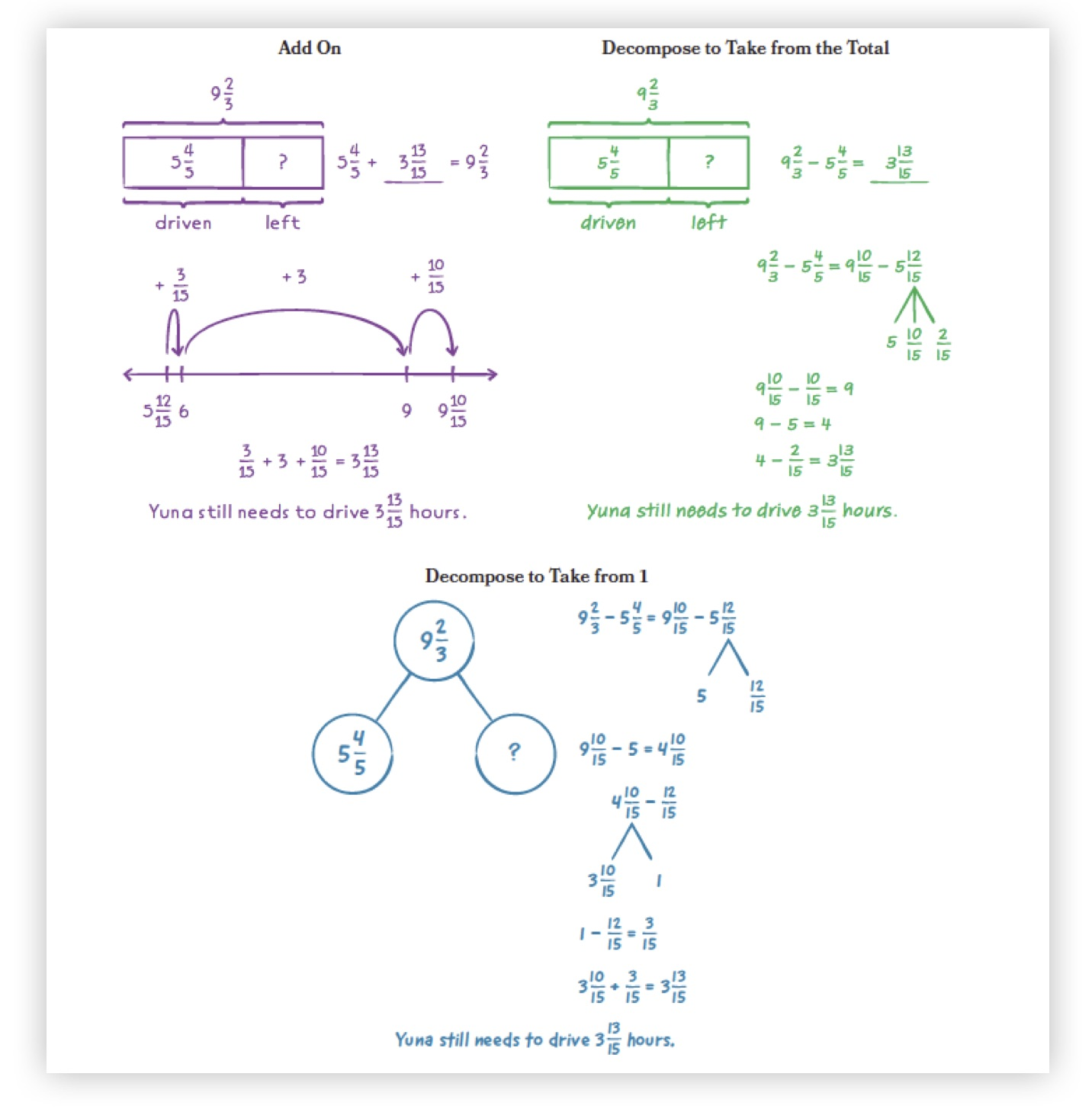Novice teachers will appreciate these possible solution paths, while their more seasoned colleagues may find their own planning validated by the examples. Teachers can use the strategies to make a chart of potential solution methods, completing this first practice and preparing them for the second practice, monitoring, which we’ll look at in the next article in this series.

## 2. Monitoring

This is the second article in a series about how creating rich, student-centered discussions in Eureka Math2 follows the principles in the book Five Practices for Orchestrating Productive Mathematics Discussions by Margaret S. Smith and Mary Kay Stein. Their five research-based practices shown below represent a unified teaching sequence of careful planning, thoughtful observation, and logical presentation of student work.

In the first practice, anticipating, teachers read about the math in the lesson they are about to teach and consider the different solution paths students might take, creating a chart to summarize those paths. This work happens before the lesson begins, and the rest of the practices occur during a lesson that’s structured around a complex, accessible instructional task. The structure of this lesson, the authors point out, typically contains phases to launch, explore, and then come together to summarize the learning (Smith and Stein 2011, 1). If that sounds familiar, it should—the Launch, Learn, Land instructional design of Eureka Math2 lessons lends itself to this mixture of collaborative work and whole-class discussion around complex mathematical tasks.

Because monitoring occurs before the whole-class discussion, the teacher has a chance to shape that student discourse in advance. This happens in two important ways: collecting information about students’ solution paths and asking questions that help students navigate and explain their chosen strategy. Understanding which students are using which strategies will help teachers with selecting and sequencing, the next two practices, so the authors recommend that teachers record this information in a simple three-column chart that they began in the first practice:

 Strategy Who and What Order

During the anticipating practice, teachers recorded as many of the solution strategies as they could imagine in the Strategy column, adding an “Other” line for strategies that they didn’t anticipate. In the monitoring practice, the teacher notes which students or groups are using each strategy in the second column, along with some quick notes about how they applied the strategy to the problem. We will see how those notes are used in the next two articles.

The practice of monitoring also allows the teacher to affect the upcoming discussion in a different way, by asking probing questions to learn more about students’ thinking or to guide them onto a more productive solution path. Learning more about how a student is thinking about solving a problem is essential formative assessment feedback. It’s also useful information for the teacher to bring up again during the whole-class discussion to highlight the thinking behind certain strategies. Asking questions before the discussion gives students a chance to revise their answers (Smith and Stein 2011, 10), allowing everyone to participate comfortably.

Asking students the right guiding questions is a delicate art. Smith and Stein explain that good monitoring questions can guide students away from an “unproductive or inaccurate pathway” or encourage other students to think more deeply about their strategies (Smith and Stein 2011, 37). But questions that require the teacher to provide the correct answer or that merely provide hints won’t generate student-focused deep thinking and rich classroom discussion (Smith and Stein 2011, 62). That’s why chapter 6 of Five Practices provides great guidelines on asking questions that strike the right balance between teacher authority and student autonomy (Smith and Stein 2011, 61–74).

Eureka Math2 provides assistance on cra¨ing questions for the monitoring practice, which begins when students start to work on the complex mathematical task, typically during the Learn segment of a lesson. Along with guidance for the next practice, selecting, this excerpt from grade 5 module 1 lesson 7 offers some suggestions for advancing questions that teachers can use to uncover their students’ thinking (Smith, Steele, and Raith 2017):

Circulate and observe student work. Select two or three students to share in the next segment. Look for work samples that help advance the lesson's objective of exploring familiar multiplication methods. Use the follow questions and prompts to elicit student thinking:

• What does this number represent? (Gesture to a number shown in the student's work.)

As part of the practice of anticipating, teachers considered how to address a solution strategy when they encounter it, including how to guide students toward applying the strategy appropriately. To help teachers with both anticipating and monitoring, Eureka Math2 includes descriptions of alternative solution paths, along with suggestions of how to respond to different approaches. This can occur in sample student dialogue and teacher directions, as shown in the excerpt below from grade 5 module 2 lesson 10:

Ask the following question to students who shared their solutions.

Why did you decide to add like units?
When I renamed the fractions, I noticed the sum of the fractions is less than 1. So, I thought it would be more efficient to add the whole numbers and then the fractions because would not need to regroup or rename.

Validate methods other students may have selected by acknowledging there are many ways to add. For example, some students may have decomposed one of the addends as 1225 + 3 + 15 before finding the sum. Encourage students to reflect on their choice of how to add for problem 1, and ask them to continue to reflect on their choices as they move through this and subsequent lessons.

Margin notes also contain valuable information about alternative strategies and how to encourage students toward the most productive solution paths.

##### Teacher Note

After they find the value of the unknown, students often lose track of what the quantity refers to within the problem context.

When they write a sentence to answer the question, it helps students build the habit of recontextualizing their work. Recontextualizing prompts students to think about whether their answer makes sense in terms of the problem and the units.

Teacher notes like this show a common student error that teachers can watch for
during monitoring.

##### UDL: Engagement

Consider providing feedback throughout the lesson that emphasizes individual effort, encourages setting goals for improvement, and acknowledges the value of finding and correcting errors. Use feedback such as the following:

• Your effort really shows here when you correctly labeled the total in your tape diagram.
• You partitioned the tape correctly and identified the unknown part with a question mark. That really shows your effort.
• Let's talk about what you can do next time to be sure you have the correct number of parts shown in your tape.

Here, teachers have some positive language they can use to help students persevere through challenges and continue to struggle productively

##### UDL: Action & Expression

Consider posting guiding questions such as the following that encourage partners to monitor and evaluate their progress as they complete problems 2-4.

Monitor

• Should we try something else?

Evaluate

• What worked well?
• What might we do differently next time?

These questions can help guide students to find solution paths and develop important metacognitive skills

Eureka Math2 can help when monitoring occurs in person, and the digital lessons support teachers with monitoring student progress in digital lessons, even when students are working remotely. In the digital lessons, teachers can watch students work in real time, which can allow students to work independently if they are in remote classrooms and permit the teacher to monitor their work efficiently and unobtrusively. Without checking individually with student groups, teachers can quickly assess which students in a group need the most help and adapt their monitoring accordingly.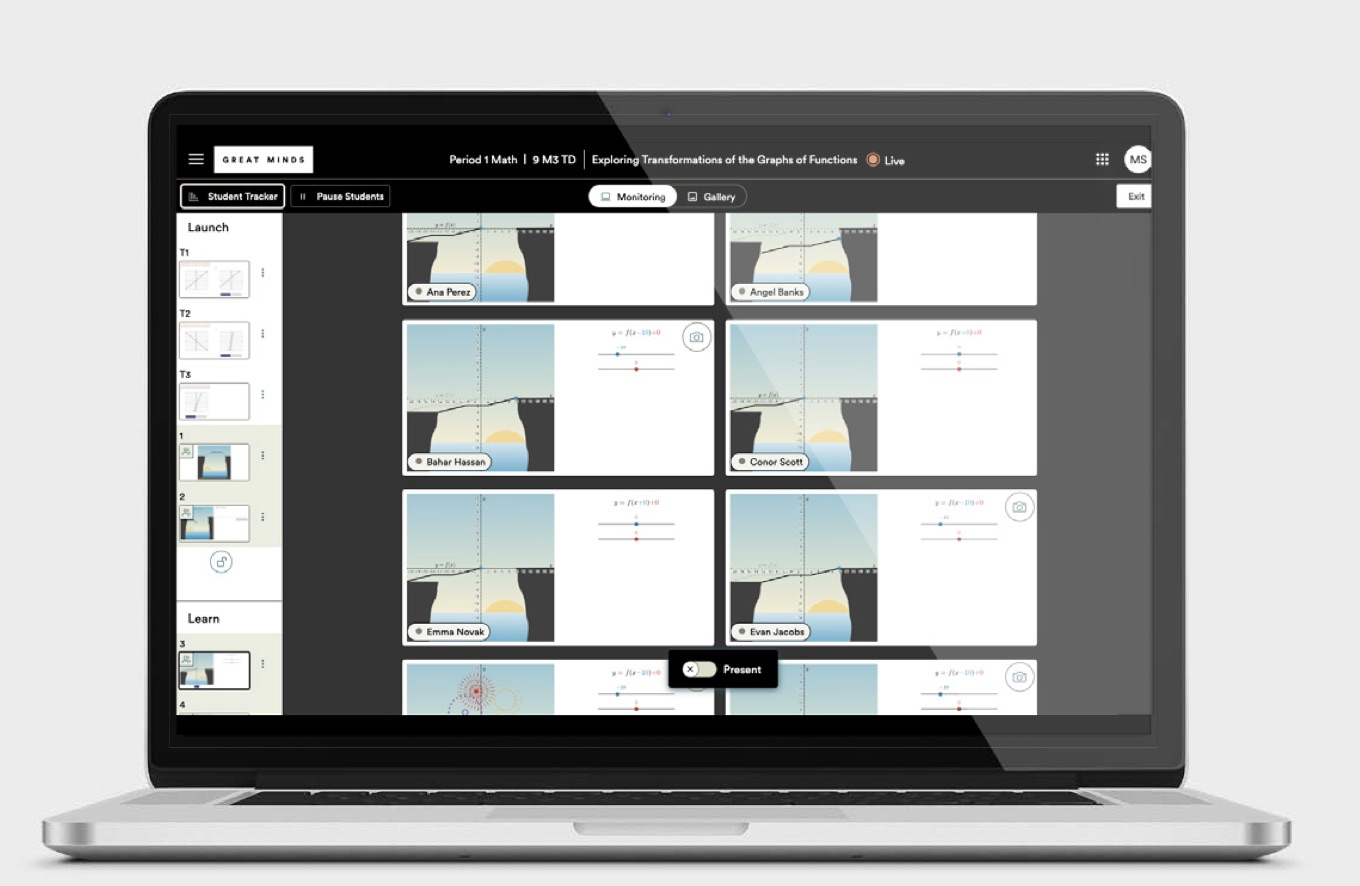The digital platform includes two important tools to support monitoring. In Monitoring mode, teachers can see and assess how students are approaching the problem on the slide.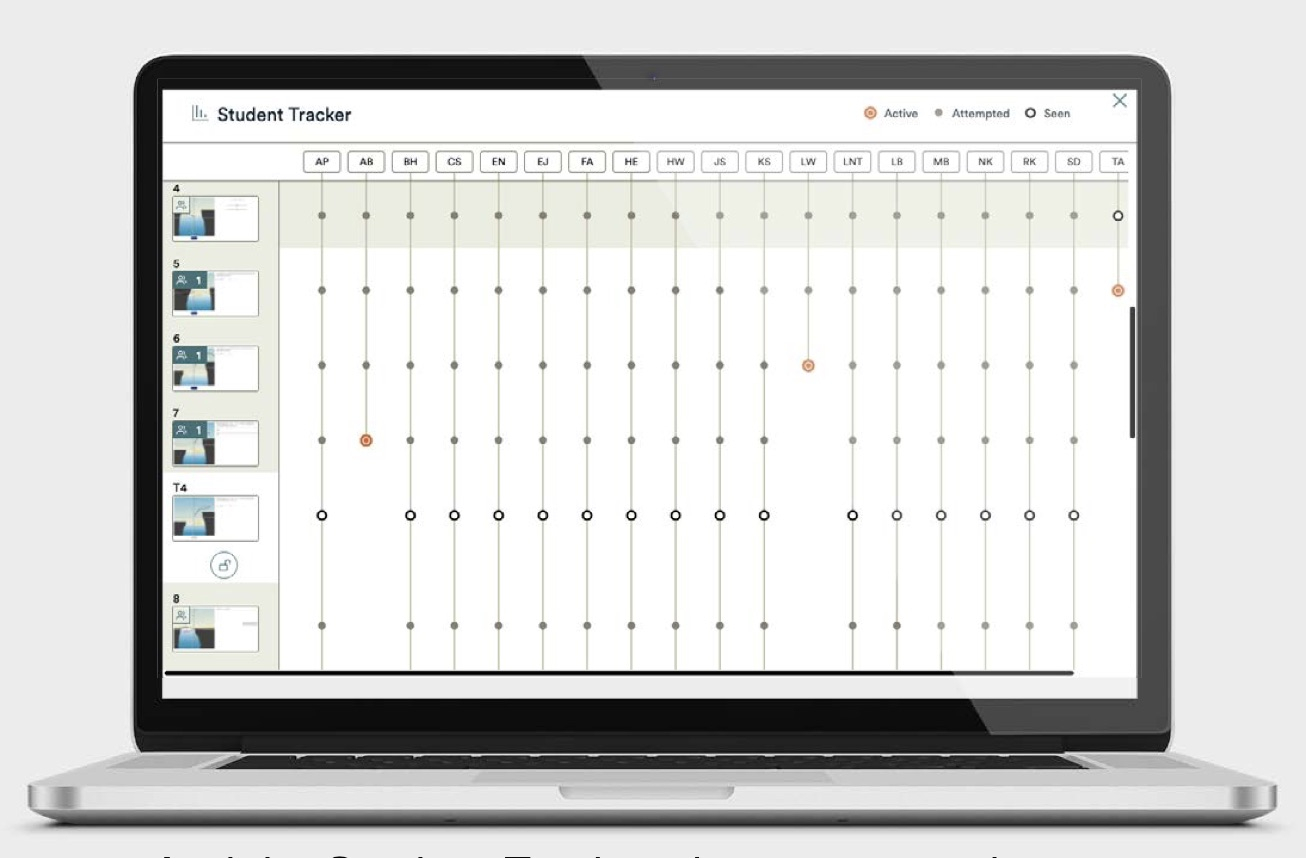And the Student Tracker shows an overview of where students are in all of the slides, so teachers can spot students who are stuck or working slower than others.

Whether the class is meeting in person or on the digital platform, Eureka Math2 makes the monitoring practice effortless and prepares teachers for the practice of selecting, which we’ll look at in the next article in this series.

## 3. Selecting

This is the third article in a series about how creating rich, student-centered discussions in Eureka Math2 follows the principles in the book Five Practices for Orchestrating Productive Mathematics Discussions (2011), by Margaret S. Smith and Mary Kay Stein. Their five research-based practices, shown below, represent a unified teaching sequence of careful planning, thoughtful observation, and logical presentation of student work.

In the first two articles in this series, we explored how Eureka Math2 helps teachers with the practices of anticipating and monitoring student work. First, teachers anticipate possible solution paths and how to respond to them. Then they monitor the student work during class, both to guide students in finding appropriate solutions and to select student work for discussion, which is the third practice.

Although it may appear shorter than the other practices in the book, selecting requires preparation and represents a crucial component of orchestrating productive class discussions. As the authors point out, thoughtfully choosing student work to share allows the teacher to keep student discussion moving toward the main ideas of the lesson.

Teachers who call on student volunteers to present their solutions are forced to think on their feet. Sometimes this means trying to figure out an unexpected solution path. Other times, they risk confusion by overwhelming students with so many solution paths that they bury the point of the lesson. By choosing work purposefully, the teacher can move the discussion in a productive direction toward the lesson goal. Making a conscious selection in advance also benefits equity, since the teacher can call on a student who might not otherwise volunteer, or who might be slower to gather their courage to raise a hand (Smith and Stein 2011, 44).

Smith and Stein offer an example of a second grade classroom where the typical “Who wants to present next?” teaching strategy went awry. As various student volunteers shared correct approaches of counting by twos, the progression of ideas was suddenly derailed by a student volunteer who offered a solution that was an odd number. As she paused discussion to determine the origin of the error, the class became focused on one student’s answer, rather than the original objective of counting by twos.

The class was left confused about the main idea of the lesson. Had the teacher monitored and selected student answers in advance, she could have guided the student privately to a be‘er solution and not asked him to present his work, thus moving the class discussion toward its conclusion (Smith and Stein 2011, 44). Choosing student work thoughtfully is essential in ensuring the class discussion progresses logically toward the main idea of the class.

Teachers can make even more thoughtful choices if they have made the chart of student strategies that the authors recommended during the first practice. Here is what that chart might look like after the teacher has observed a few students.

 Strategy Who and What Order Tape diagram Shen—annotated with incorrect unitsBlake—grouped correctly Area model Leo—unique arrangementRiley—equation error Equation Kayla—showed all steps clearly

During the practice of selecting, teachers can fill out the second column with the student’s name along with any notes about how the students are using this strategy. This method takes some preparation, however, and Eureka Math2 and its digital tools make the selecting practice easier in several ways.

Teach embeds guidance in lessons to help teachers know what kinds of examples to look for—and even what to do if they’re not seeing any students use a particular solution strategy. These explanations often cover more than just one practice, like the examples below from lessons in grade 5 module 2. They tell teachers what to anticipate and select, and they provide us with some foreshadowing for the last two practices of sequencing and connecting. This highlights how interconnected the five practices are, and how it’s often hard to talk about one practice in isolation.

##### Teacher Note

The sample student work and student thinking show common responses. Look for similar work from your students and encourage authentic conversations about the key concepts.

If your students do not produce similar work, choose one or two pieces of their work to share and highlight how it shows movement toward the goal of the lesson. Then select one sample work from the lesson that would best advance student thinking. Consider presenting the work by saying, "This is how another student solved the problem. What do you think this student did?"

Circulate and monitor student work. Look for students who approach the models differently. Expect some students to draw circles to represent the friends and then divide each sticky note into fourths, while other students may distribute 4 whole sticky notes among the friends first.

When teachers are using digital lessons, they can easily view and select examples of their students’ work. Once the student completes their work, the teacher can get a frame capture of the work and save it to their Gallery view for later organization and presentation.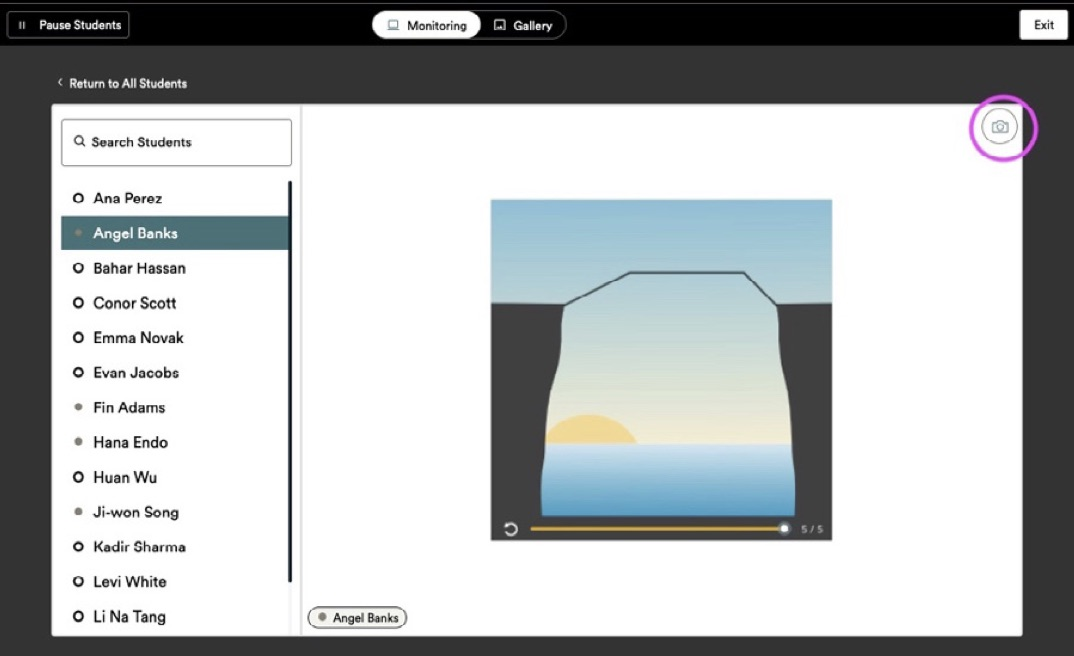Up to four frame captures can be added to a slide, or they can be shown individually in any order the teacher chooses.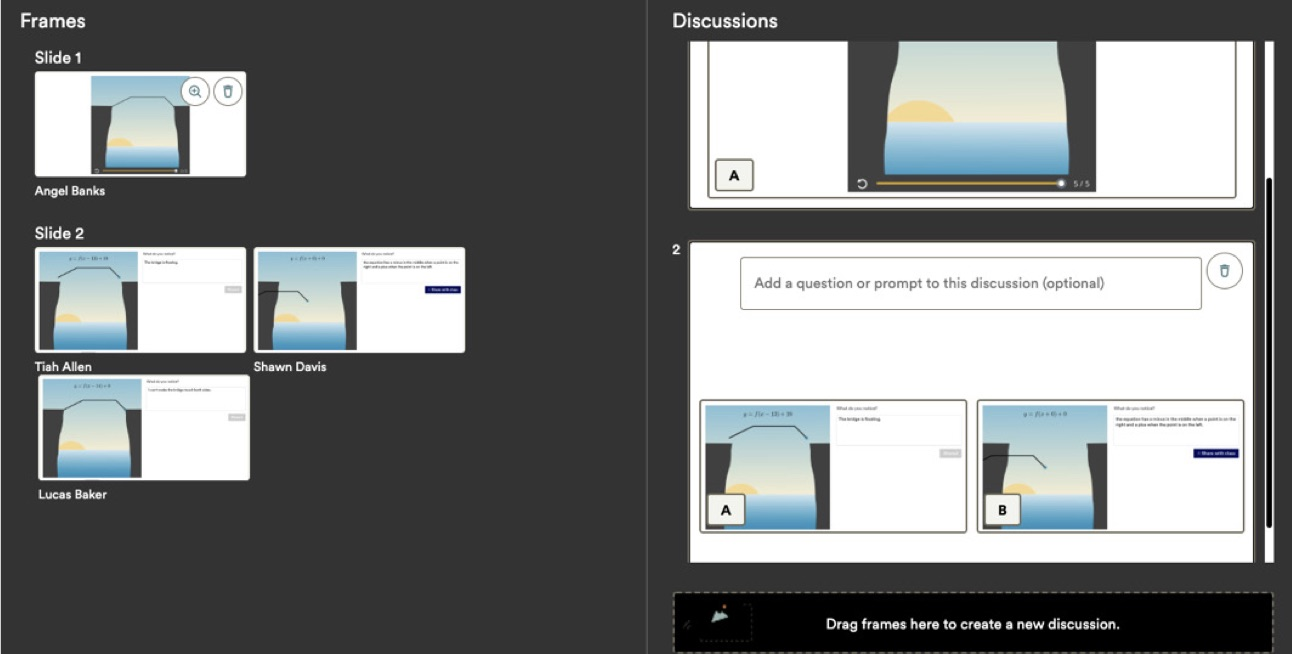Using these Presentation Tools allows the teacher to easily work from a list of strategies they want to discuss with the class. One added advantage of seeing the whole class together is that the teacher can quickly recognize how many students are using each strategy and then decide whether to highlight variations of a popular solution path or focus on an interesting method the few have used.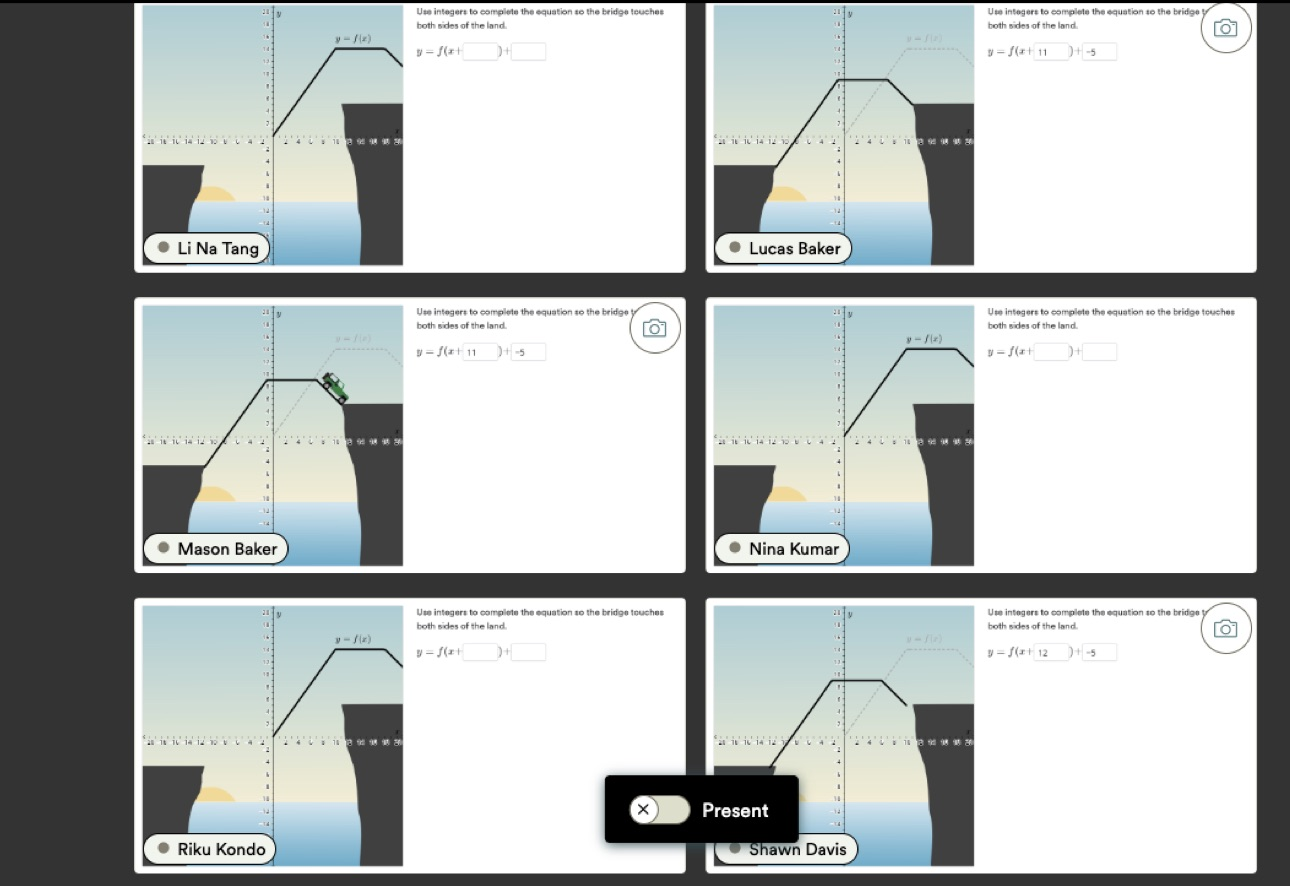Choosing the right work is an important part of inspiring classroom discussion, but what teachers do with those work examples is essential to drive math discourse and support the goal of the lesson. In the next two articles, we'll look at the final two steps in creating productive classroom discussions.

## 4. Sequencing

This is the fourth article in a series about how creating rich, student-centered discussions in Eureka Math2 follows the principles in the book Five Practices for Orchestrating Productive Mathematics Discussions (2011), by Margaret S. Smith and Mary Kay Stein. Their five research-based practices, shown below, represent a unified teaching sequence of careful planning, thoughtful observation, and logical presentation of student work.

The first three articles in this series have shown how Eureka Math2 helps teachers anticipate, monitor, and select student work. First, teachers anticipate possible solution paths and how to respond to them, and then they monitor students engaging in the task during class and select student work to discuss. Once they’ve selected the work, they follow the fourth practice and arrange it in a deliberate order that strategically builds to the underlying mathematical concepts.

Smith and Stein explain that sequencing accomplishes two goals: improving the accessibility of the mathematics and creating a logical flow to the presentation of student work (Smith and Stein 2011, 11, 44). By presenting a straightforward example first, teachers can help make connections to more complex or unexpected solution paths. Once this entry point is chosen, sequencing of student work is a ma‘er of deciding how to build to the lesson’s desired mathematical goals. As the authors phrase it, sequencing reflects how the teacher wants “to build a mathematically coherent story line” (Smith and Stein 2011, 44).

Just as there are many ways to tell a story, there is no one way to sequence student responses (Smith and Stein 2011, 49). In general, teachers should start with the most accessible strategies and proceed toward more complex and abstract ones. However, the decision depends on a teacher’s style and the makeup of their class. For a class familiar with using tape diagrams for division, problems might begin with that representation to ease into a new concept. Teachers can start by highlighting a common error or an innovative approach from a student—or save that innovation for last.

No matter what sequence they choose, the order is going to change for each class and each group of students. Each time a teacher sequences responses, it’s an opportunity to tell the story in a different way, with the voices of their students, not the teacher, as narrator. And this is the secret power of sequencing: guiding students to tell that story in their own way. When students explain their mathematical understanding in a way that makes sense to them, they grasp the concepts more deeply and more clearly. And when they hear their classmates explain the ideas in their own way and then respond to those explanations, they not only hear their classmates stories but also learn about cooperation, argumentation, and reasoning (Chapin, O’Connor, and Anderson 2009, 7).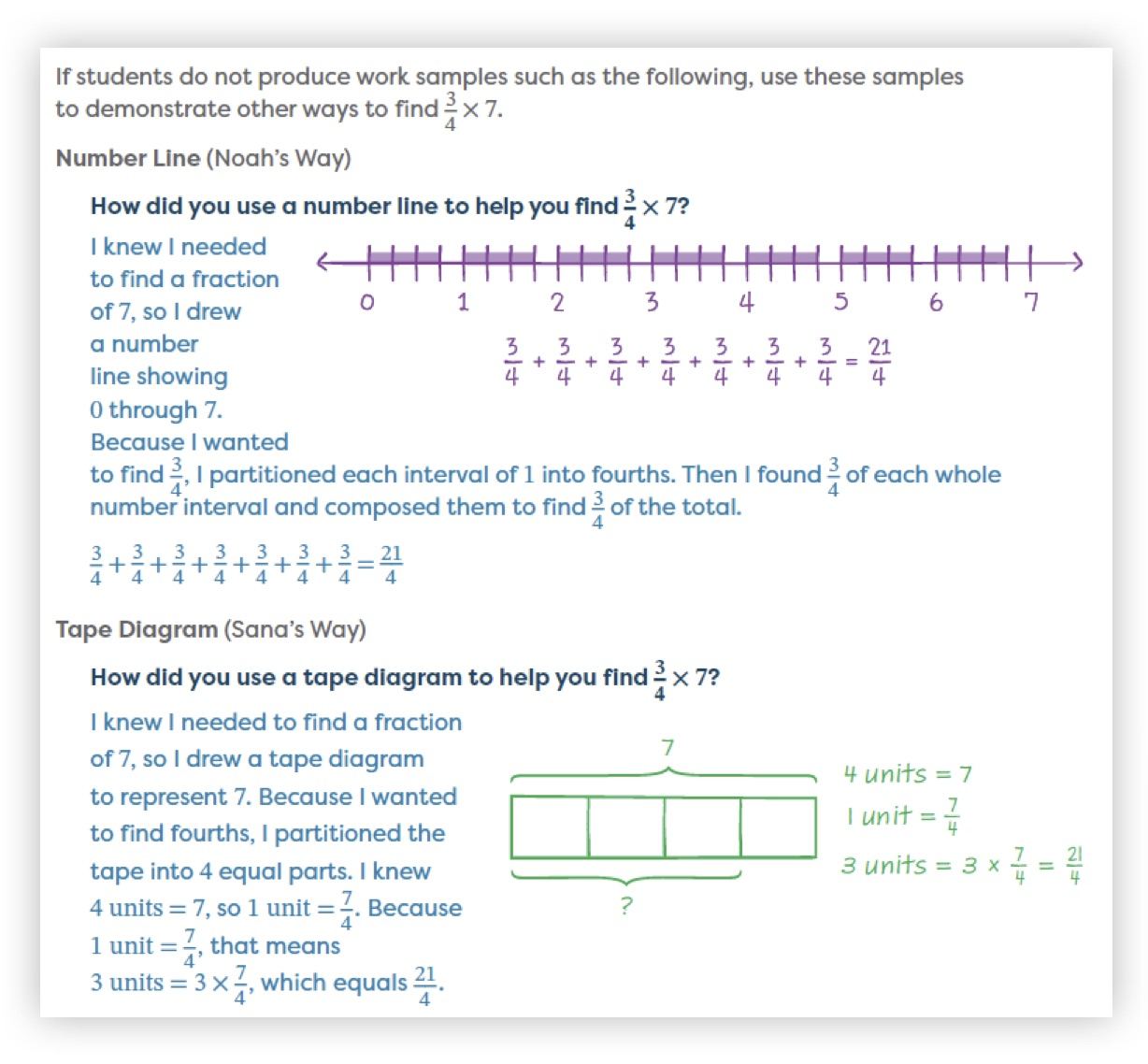Because so much of sequencing is individual to your class and approach, Eureka Math2 doesn’t offer specific suggestions about student work sequences. However, lessons present student work by using sample solutions that are arranged from accessible to complex. In grade 5 module 3 lesson 4, the problem is represented in the first example with a number line, in the second with a tape diagram, and the third with an equation. The methods become increasingly abstract and illustrate how well-sequenced representations lead to equations for a deep understanding of the underlying concepts.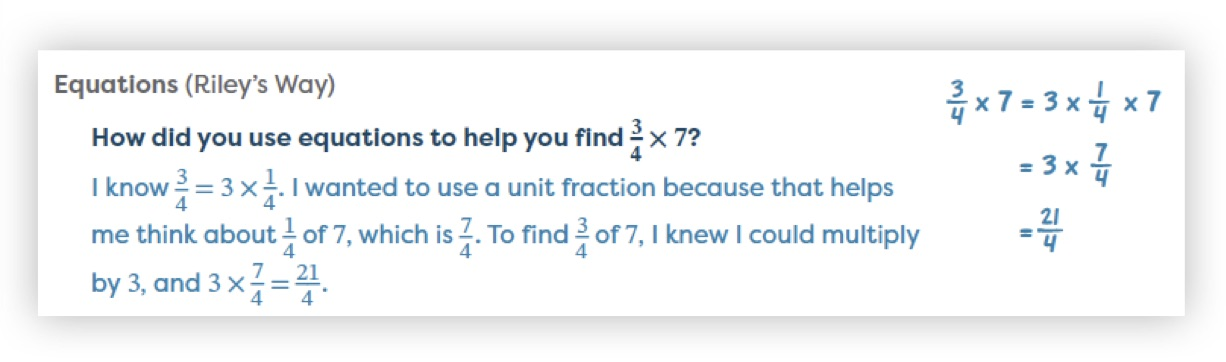In digital lessons, the Presentation Tools allow teachers to thoughtfully sequence the student work they present. After capturing student work, shown in the Frames section, teachers can then drag and drop student responses into the Discussions section in the order they prefer, with up to four examples on a slide, and an optional title. Clicking Present allows teachers to start showing their sequenced student work and build great discussions.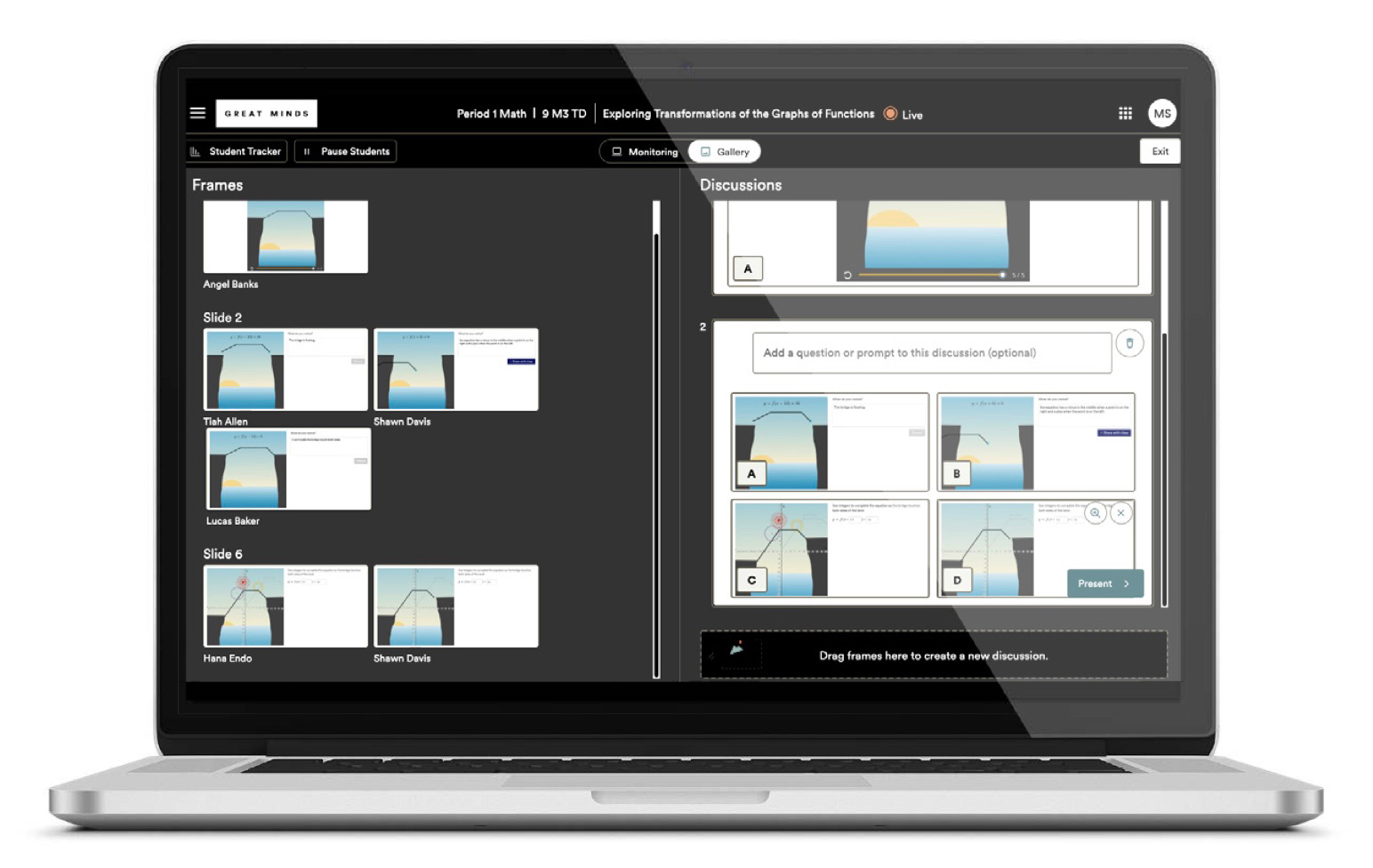Just as Eureka Math2 tells a mathematical story across and within modules, sequencing student work allows teachers to extend that same story in their lessons, or to tell a different one. The crucial step of sequencing transforms a “show and tell” class discussion, where student work is presented in any order and without a clear point, into a discussion that is focused on the lesson objective and the larger mathematical goals. And teachers can accomplish this without seeming to guide the discussion at all, giving their students the crucial agency to take control of their mathematical thinking.

Though it might seem like the orchestration of productive mathematical discussion is now complete, there’s one more essential step to bring it all home and build mathematical knowledge effectively—and that’s covered in the last article in this series.

## 5. Connecting

This is the fifth article in a series about how creating rich, student-centered discussions in Eureka Math2 follows the principles in the book Five Practices for Orchestrating Productive Mathematics Discussions (2011) by Margaret S. Smith and Mary Kay Stein. Their five research-based practices, shown below, represent a unified teaching sequence of careful planning, thoughtful observation, and logical presentation of student work.

The first four articles in this series have explained how Eureka Math2 helps teachers anticipate, monitor, select, and sequence student work. First, teachers anticipate possible solution paths and how to respond to them, and then they monitor the student work during class and select student work to discuss. They bring the work into a discussion in a specific order that builds to important concepts, and in the final practice, teachers establish connections to make these concepts explicit.

These connections show how the different student work examples relate to one another, to concepts students have previously learned, and to the new concepts they are learning. Consistent with the underlying social constructivist theory, however, teachers can’t just tell students what these connections are. They need to ask students the right questions so that students can discuss and understand the ideas themselves. And that, Smith and Stein (2011) point out, makes this the hardest practice of all (49).

The questions are so difficult to formulate because they must be grounded in the students’ work to be effective, and they must start with what students already know (Smith and Stein 2011, 50). If that sounds like a lot to pack into a question, it is. And that’s why every Land segment of every Eureka Math2 lesson has already formulated these questions for the teacher.

The Debrief for grade 7 module 3 lesson 17 shows how the questions invite students to connect familiar concepts—such as proportional relationships, equivalent ratios, and if–then moves for solving equations—with new learning: equations in the form a/b = c/d.

Debrief 5 min

Objective: Write and solve equations in the form ab = cd, where either a, b, c, or d is unknown and the other three are specific rational numbers.

Use the following prompts to guide a discussion about equations in the form ab = cd.

When can we use an equation in the form ab = cd?
Equations in that form are used to represent proportional relationships.

How can we solve an equation in the form ab = cd
Sometimes we can solve equations in that form just as we would solve other equations, by using if-then moves. Otherwise, we can create equivalent ratios to find the unknown value.

When is it helpful to use an equation in the form ab = cd?
When you know that a relationship is proportional but do not know the constant of proportionality, you can write an equation in this form.

If a teacher isn’t sure what foundational knowledge students need, or if they want to help students make further connections, Eureka Math2 has just what they need. The curriculum makes these connections throughout the Teacher Edition, starting at the module and topic levels before narrowing to the lesson and conceptual levels. Let’s look at how this works in grade 7 module 3 lesson 17, the lesson with the Debrief above.

At the beginning of each Eureka Math2 module, a sidebar called Before This Module shows the concepts from previous modules that are built upon in this module.

##### Before This Module

In grade 6, students apply properties of operations to solve one-step equations of the forms x + p = q and px = q for cases in which p, q, and x are nonnegative rational numbers. In this module, students extend this work to negative rational numbers and to the forms px + q = r and p(x + q) = r. In grade 6, students write inequalities of the form x > c or x < c to represent a constraint in a problem. Such inequalities have infinitely many solutions that can be represented on a number line. In this module, students extend that learning to include solving inequalities of the form px + q > r or px + q < r, where p, q, and r are specific rational numbers. Students solve inequalities that are less than or equal to and greater than or equal to and interpret the solution set in the context of the problem.

Then the Topic Overview outlines the major concepts of the module and how they build on each other. Here’s part of the Topic C narrative:

In topic B, students begin applying if-then moves to solve equations that represent angle relationships. Students continue to use if-then moves in topic C to solve equations of the forms px + q = r and p(x + q) = r, where p, q, and r are specific rational numbers. Students also continue to use if-then moves to solve equations of the form ab = cd, where a, b, c, or d is unknown and the other three are specific rational numbers.

The lessons themselves contain many connections that teachers can make explicit to their students, starting with the Lesson at a Glance in the beginning. Here’s what that section looks like for Lesson 17:

Lesson at a Glance

In this lesson, students combine what they already know about proportional relationships and equations to explore a new type of equation in the form ab = cd. Students explore multiple ways to write these equations from proportional relationships. Then students discover two strategies for solving equations in the form ab = cd: by using if-then moves and by using equivalent values of the ratio. Pairs of students examine relationships to determine whether they are proportional. If they are, students write and solve an equation in the form ab = cd to find an unknown value in the relationship.

In this lesson, students are asked to contrast different strategies as a way of seeing the connections between the two solution paths.

 14⁄2 = x⁄8 2⁄14 = 8⁄x

Facilitate a discussion by using the following prompts.

Why did you write your equation like that?
In problem 2, we found the constant of proportionality by dividing the number of mini muffins by the number of cups of flour, so I wrote 142.
In this problem, 2 came first, so I wrote it as 214

Raise your hand if you wrote the equation 142 = x8. How did you find the value of x?
I used an if-then move by multiplying both sides of the equation by 8.
I multiplied 142 by 44.

Would those strategies work for the equation 214 = 8x?
Since the variable is in the denominator instead of the numerator, I can't multiply both sides by 8.
I can multiply 214 by 44 to determine 56 was the value of x.

Can we write the equation as 214 = 8x?
Yes. We were able to find the same value of x.

We just had to use different strategies. Since the relationship of 2 cups of flour needed for 14 muffins is the same as the relationship of 14 muffins needing 2 cups of flour, we can write the equation either way. If the variable is in the denominator, we just have to use different strategies to solve, but it is possible.

These questions are samples of what could be asked based on these specific examples, but the questions illustrate how making connections in classroom discussions affect students. As students are asked about their own work and the work of others, they consider the choices they made and defend the logic behind them. They see their own thinking validated by the teacher, even if the thinking is flawed or applies in a different context, and they gain clarity and self-confidence as they make sense of the math with their peers. Eureka Math2 demonstrates the approaches in every lesson with these example discussions, anchoring them in the students’ work and explaining concepts, both to the teacher and to the student.

The activities in digital lessons provide another dimension to the connecting step by allowing students to connect new learning with their existing knowledge. For example, lesson 22 in grade 6 module 1 helps students make connections between percents and the familiar representation of their device’s battery level.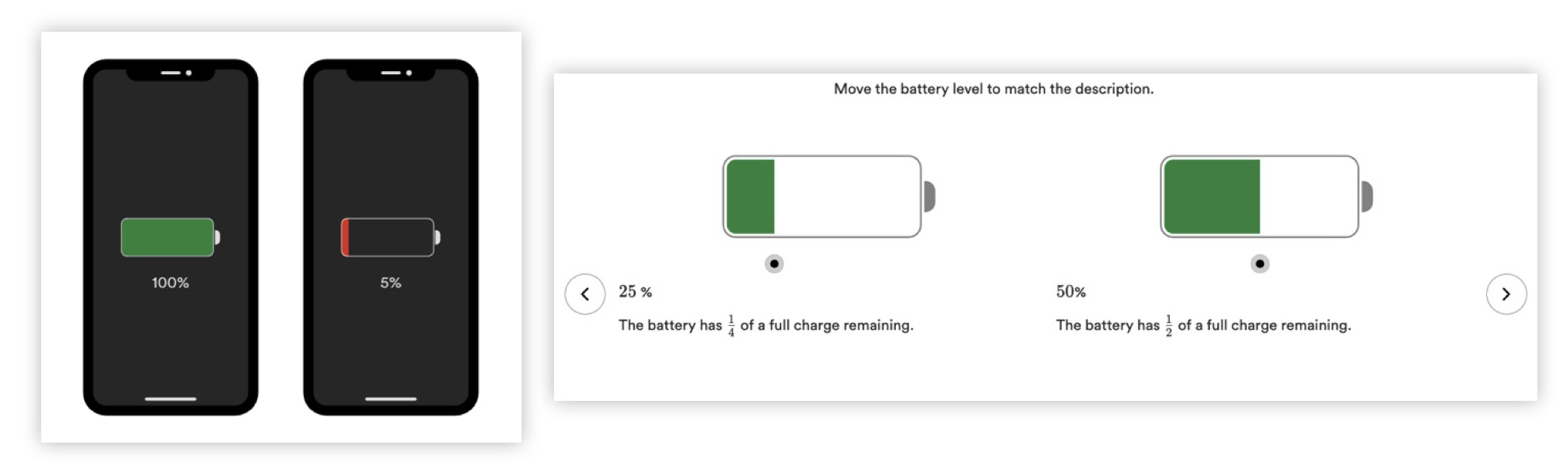Whatever ideas teachers want to connect can be easily highlighted in digital lessons by the teacher’s careful choice and sequence of student work with the Presentation Tools. As they select student work, teachers have to think about how the work expresses and connects to important mathematical concepts that thread through students’ mathematical journeys. And because the Eureka Math2 text makes so many of these connections explicit and explains them, it’s so much easier to see how student work illustrates these concepts.

Beyond mathematical learning and achievement, productive classroom discussions can build many other skills, like collaboration, self-confidence, and argumentation (Chapin, O’Connor, and Anderson 2009, 8–9, 165–7). Discussions like these help students to write their own mathematical story, to see math through many different lenses, and to think about themselves and the world around them in a completely different way. There are so many reasons for teachers to build these essential classroom discussions, and the five practices will help teachers conduct them effectively, with plenty of support throughout Eureka Math2 and its powerful, versatile digital platform.

Carpenter, Thomas P., Elizabeth Fennema, Megan Loef Franke, Linda Levi, and Susan B. Empson. 2014. Children’s Mathematics: Cognitively Guided Instruction, 2nd ed. Portsmouth, NH: Heinemann.

Chapin, Suzanne H., Catherine O’Connor, and Nancy Canavan Anderson. 2009. Classroom Discussions: Using Math Talk to Help Students Learn, Grades K–6. Sausalito, CA: Math Solutions.

Kuehnert, Eloise R. A., Colleen M. Eddy, Daphyne Miller, Sarah S. Pra‘, and Chanika Senawongsa. 2018. “Bansho: Visually Sequencing Mathematical Ideas.” Teaching Children Mathematics 24, no. 6 (2018): 362–69. https://doi.org/10.5951/teacchilmath.24.6.0362.

Smith, Margaret S., and Mary Kay Stein. 2011. Five Practices for Orchestrating Productive Mathematics Discussions. Reston, VA: National Council of Teachers of Mathematics.

Smith, Margaret S., Michael Steele, and Mary Lynn Raith. 2017. Taking Action: Implementing Eective Mathematics Teaching Practices in Grades 6–8. Reston, VA: National Council of Teachers of Mathematics.

Vadeboncoeur, Jennifer A., and Louai Rahal. “Sociocultural Approaches to Learning and Development.” 2013. In Sociology of Education: An A-to-Z Guide, edited by James Ainsworth, 720–23. Thousand Oaks, CA: SAGE Publications, Inc., 2013. hps://dx.doi.org/10.4135/9781452276151.n378.